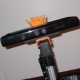ROS Resources: Documentation | Support | Discussion Forum | Index | Service Status | Q&A answers.ros.org

# openni_camera depth image opencv

Hi

I am Using opencv 2.2 and cv::bridge out of the ros diamondback deb packages. I changed the Makefile of opencv to get a newer version of opencv (Revision 5427)

I convert the const sensor_msgs::ImageConstPt to cv_bridge::CvImagePtr with

cv_ptr_depth_ = cv_bridge::toCvCopy(depth_image_msgs, sensor_msgs::image_encodings::TYPE_32FC1);


With cv::VideoWriter I want to store the depth image as an avi file. That works fine for the rgb image that is converted to sensor_msgs::image_encodings::BGR8 but not for the depth image. The cv::VideoWriter needs images with depth = IPL_DEPTH_8U and nChannels = 1.

But I cannot convert the 32FC1 type in 8UC1

How can I handle this problem?

Thanks Stefan

edit retag close merge delete

Sort by » oldest newest most voted

Hi Stefan,

There might be a more direct answer to your question, but Mike Ferguson has a depth_viewer ROS package that does the conversion I think you need. Check it out at http://www.ros.org/wiki/depth_viewer.

--patrick

moreFirst up is understanding the format of the message. It is indeed a 32FC1 -- meaning it is a 32-bit float. This float corresponds to depth in meters (which concurs with ROS standards).

If you then need to convert to an 8UC1 image, you can scale the 32-bit float into the min->max range (something like 0.0 or 0.5m to 5.5m):

    cv::Mat img(cv_ptr_depth_->image.rows, cv_ptr_depth_->image.cols, CV_8UC1);
for(int i = 0; i < cv_ptr_depth_->image.rows; i++)
{
float* Di = cv_ptr_depth_->image.ptr<float>(i);
char* Ii = img.ptr<char>(i);
for(int j = 0; j < cv_ptr_depth_->image.cols; j++)
{
Ii[j] = (char) (255*((Di[j]-min_range_)/(max_range_-min_range_)));
}
}


Where min_range_ and max_range_ would be floats, set to your min and max range. This also means you'll be able to actually view data with the human eye (the float32 representation generally just looks black, since the numbers are all fairly small).

This same block of code does show up in the depth_viewer I wrote, as Patrick mentioned.

more

I think we can use this code , it is more efficient than the above code double minVal, maxVal; minMaxLoc(cv_ptr->image, &minVal, &maxVal); //find minimum and maximum intensities //Mat draw; cv_ptr->image.convertTo(blur_img, CV_8U, 255.0/(maxVal - minVal), -minVal * 255.0/(maxVal - minVal));

I want disparity in pixels .... Can you suggest how can I modify your code accordingly :) Thanks in advance :)

Hi Mike, hi Patrick

That works fine for me but now I have a new problem. I want to save the depth image as an video with the VideoWriter. I initialize it as follows:

cv::VideoWriter depth_writer_;

depth_writer_.open("/home/stefan/Desktop/depth_video.avi", CV_FOURCC('D','I','V','3'), 20, cv::Size(640,480),false);
if( !depth_writer_.isOpened())
ROS_ERROR("[KinectTracking::KinectTracking] DEPTH failed to open!\n");


that works as well fine with me but the output is a video where three images are shown side by side. If I use the color images and set the last parameter to true that works find.

Does anybody have an idea to solve that?

Thanks a lot

Stefan

more